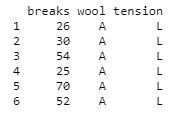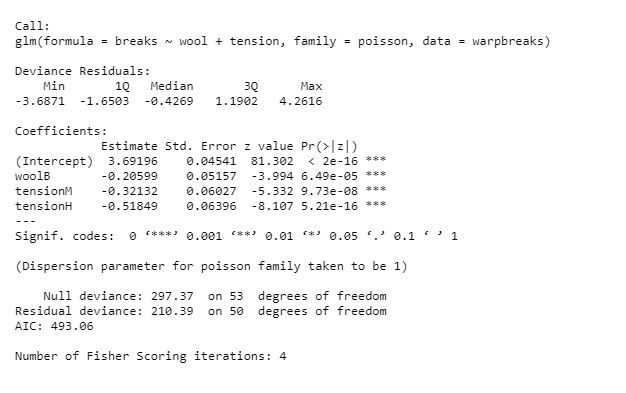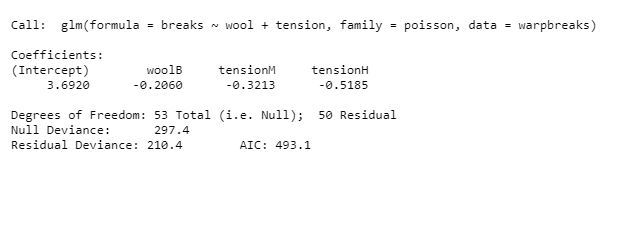Related Articles
Poisson Regression in R Programming
• Last Updated : 10 May, 2020

A Poisson Regression model is used to model count data and model response variables (Y-values) that are counts. It shows which X-values work on the Y-value and more categorically, it counts data: discrete data with non-negative integer values that count something.

In other words, it shows which explanatory variables have a notable effect on the response variable. Poisson Regression involves regression models in which the response variable is in the form of counts and not fractional numbers.

Mathematical Equation:

`log(y) = a + b1x1 + b2x2 + bnxn.....`

Parameters:

• y: This parameter sets as a response variable.
• a and b: The parameter a and b are the numeric coefficients.
• x: This parameter is the predictor variable.

#### Creating Poisson Regression Model

The function used to create the Poisson regression model is the `glm()` function.

Syntax: glm(formula, data, family)

Parameters:

• formula: This parameter is the symbol presenting the relationship between the variables.
• data: The parameter is the data set giving the values of these variables.
• family: This parameter R object to specify the details of the model. It’s value is ‘Poisson’ for Logistic Regression.

Example:

Approach: To understand how we can create:
• We use the data set “warpbreaks”.
• Considering “breaks” as the response variable.
• The wool “type” and “tension” are taken as predictor variables.

Code:

 `input` `<``-` `warpbreaks``print``(head(``input``))`

Output:#### Create Regression Model

Approach: Creating the poisson regression model:

• Take the parameters which are required to make model.
• let’s use summary() function to find the summary of the model for data analysis.

Example:

 `output <``-``glm(formula ``=` `breaks ~ wool ``+` `tension,``             ``data ``=` `warpbreaks, family ``=` `poisson)``print``(summary(output))     `

Output:#### Creating Poisson Regression Model using `glm()` function

Approach: Creating the regression model with the help of the glm() function as:

• With the help of this function, easy to make model.
• Now we draw a graph for the relation between “formula”, “data” and “family”.

Example:

 `output_result <``-``glm(formula ``=` `breaks ~ wool ``+` `tension,``                    ``data ``=` `warpbreaks, family ``=` `poisson)  ``output_result `

Output:Attention reader! Don’t stop learning now. Get hold of all the important DSA concepts with the DSA Self Paced Course at a student-friendly price and become industry ready.

My Personal Notes arrow_drop_up